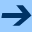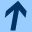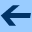Displayed equations

### Displayed equations

The LaTeX commands to format a formula as a display differ from the commands to format an inline formula only in the choice of the delimiters that identify mathematics mode. In the following example, the pair $ and $ delimit display mathematics mode.

Here is a displayed
$\int\frac{d\theta} {1+\theta^2}= \tan^{-1}\theta+C$ equation.


An equivalent way to format the same displayed equation is to spell out the delimiters in words.

Here is a displayed
\begin{displaymath}\int\frac {d\theta}{1+\theta^2}
=\tan^{-1} \theta+ C\end{displaymath}
equation.


A variant of the above is the following.

Here is a displayed
\begin{equation}\int \frac{d\theta}{1+\theta^2}=
\tan^{-1}\theta+C\end{equation} equation.


There is a difference between the displaymath environment and the equation environment: the latter automatically typesets a formula number.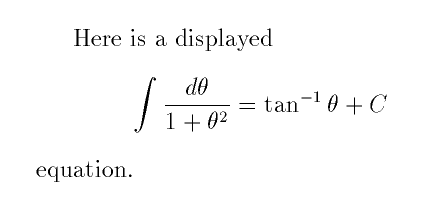The formatted output with no formula number is shown in the first figure.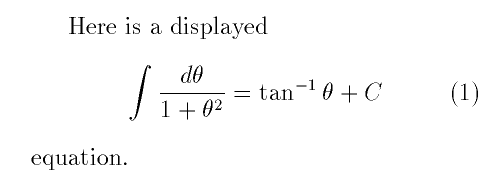The formatted output with a formula number is shown in the second figure.

Warning to former plain TeX users: in plain TeX, double dollar signs  $$ are used to delimit display mathematics, but this convention should not be used in LaTeX (although it will work sometimes). The fleqn document-class option, for example, operates by redefining $ and $, but it pays no attention to $$.

A convenient feature of LaTeX is that it can automatically generate formula numbers. If you add or delete a numbered equation, you do not have to worry about revising the formula numbers: LaTeX does this chore for you.

What if you want to refer to a numbered equation by number? How do you manage this if the equation number is automatically generated? LaTeX has a simple \label and \ref mechanism for handling symbolic cross references. Here is an example.

The formula \begin{equation} E=m c^2
\label{Einstein} \end{equation} has passed into popular culture,
but the true significance of the mass-energy
equation~(\ref{Einstein}) is~\ldots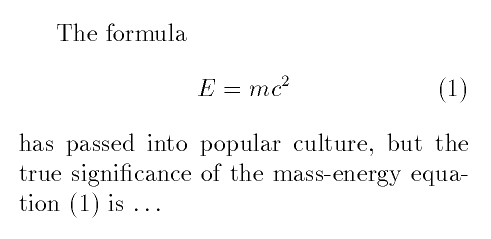(By the way, that tilde  ~ is a tie or non-breaking space: it is good typesetting style to keep a short label attached to its noun.)

If you use the amsmath package, then you can type \eqref instead of \ref, and the parentheses around the cited equation number will be supplied automatically.The Math 696 course pages were last modified April 5, 2005.Displayed equations